# What is Resistance?

## What is resistance? Learn about the fundamentals of resistance, calculation methods, and resistors

### Overview

If you’re like many people, you might have heard of resistance but don’t really understand it. That said, you may be hesitant to ask people about it now. Put simply, resistance is a force that counteracts the flow of electricity.

Resistance influences the flow of electricity. This page offers basic knowledge about resistance, along with a detailed explanation of topics such as calculation methods and resistors.

### What is resistance?

Resistance to electricity–that is, electrical resistance–is a force that counteracts the flow of current. In this way, it serves as an indicator of how difficult it is for current to flow. Resistance values are expressed in ohms (Ω).

When an electron differential exists between two terminals, electricity will flow from high to low. Resistance counteracts that flow. The greater the resistance, the lower the current. Conversely, the lower the resistance, the greater the current.

•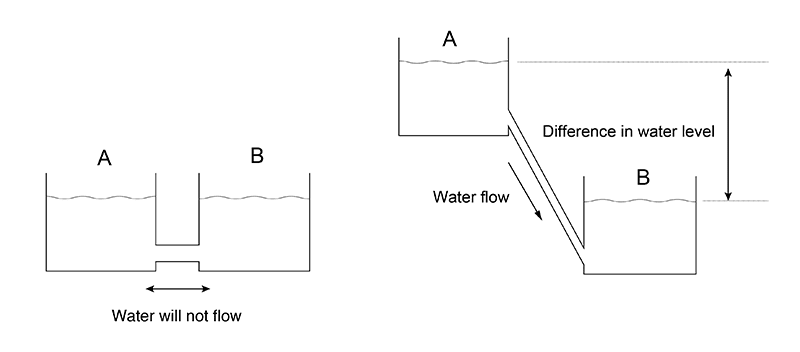### Calculating resistance

Resistance can be calculated as a value using the voltage and current in the circuit.

• Resistance = Voltage / current

This formula is known as Ohm’s Law. If voltage is held constant, the resistance value will decrease as the current–the denominator–increases. Conversely, the resistance value will increase as the current decreases. In other words, resistance is low in circuits carrying large currents, and high in circuits carrying small currents.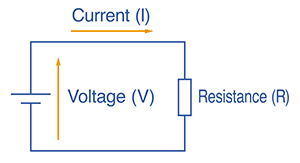In principle, resistance is determined by the type and temperature of the substance through which the electricity is flowing, as well as its length. Generally speaking, electricity flows more readily through metals due to their low electrical resistance, which varies with the type of metal, increasing in the following order: silver → copper → gold → aluminum → iron. Additionally, resistance decreases with temperature, while increasing temperature means increased resistance.

Furthermore, resistance increases with the length over which a current must travel. Conductors with a large cross-sectional area have low resistance since electricity flows more readily through them, while conductors with a small cross-sectional area have higher resistance.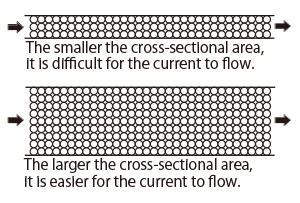The relationship between the cross-sectional area of a substance and the amount of current flowing

### What are resistors?

Resistors are electronic components that resist the flow of electricity in a circuit. Resistors are used in electric circuits to adjust current and voltage, in much the same way as faucets are used to adjust the flow of tap water. They can be used not only to control the flow of current, but also to distribute voltage in a circuit.

Electronic circuits need resistors in order to operate under appropriate conditions. Resistors are made of materials that resist the flow of electricity as it passes through them. In this way, they can control the flow of current throughout a circuit. When current is reduced by a resistor, the surplus electrical energy is converted into heat.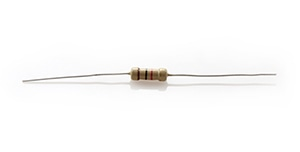Resistors

Resistors are available in a variety of types, including the following principal varieties:

• Fixed resistors
• Variable resistors
• Potentiometers

Major types of fixed resistors include carbon-film resistors and metal-film resistors, which incorporate a carbon or metal coating, respectively. These resistors have fixed resistance values. Variable resistors have resistance values that can be changed. Potentiometers are a type of variable resistor that is used to fine-tune voltage and current.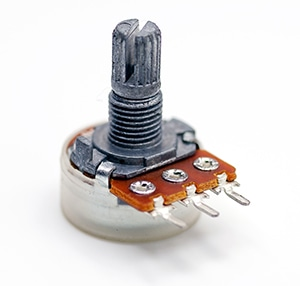Variable resistor

### Methods for measuring resistance

Resistance in a circuit can be measured using a digital multimeter. These instruments can measure not only resistance, but also voltage, current, and other parameters, making them a useful tool in a variety of situations. To use a digital multimeter, turn on the instrument and set it to resistance (Ω) mode.

Select the range as necessary based on the measurement target’s resistance value. Insert the red test lead’s plug into the “Ω” terminal and the black test lead’s plug into the COM terminal. Then place the test leads in contact with both ends of the resistor. Check the measurement result as displayed on the instrument’s LCD screen. Once measurement is complete, remove the test leads from the resistor.

•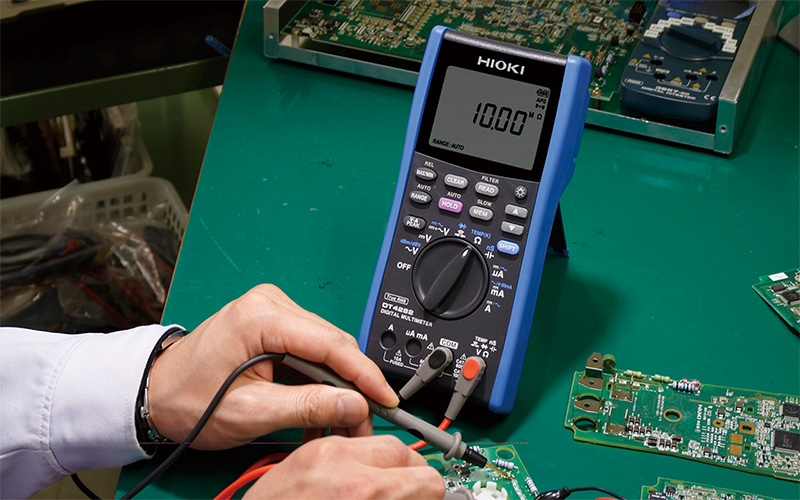Resistance is affected by a variety of factors, including temperature. Some digital multimeters provide functionality for applying correction to account for external influences, for example in the form of a resistance meter temperature conversion function. Consequently, it’s a good idea to check available models’ functionality when purchasing a digital multimeter.

### Resistors are essential in order to facilitate the appropriate flow of current.

Resistance serves as an indicator that quantifies how readily current will flow in a circuit using ohms (Ω) as the unit. Current increases when resistance decreases, and it decreases when resistance increases. Resistors are essential in order to ensure that current flows at the appropriate level in circuits. A variety of resistors are used to measure resistance in specific applications.

Digital multimeters are necessary in order to measure whether resistors are operating properly. Why not try measuring resistance while referring to the method described above?